# Origin of the Poisson Equation

In deriving the,

Poisson Equation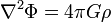$\nabla^2 \Phi = 4\pi G \rho$

we will follow closely the presentation found in §2.1 of [BT87].

According to Isaac Newton's inverse-square law of gravitation, the acceleration,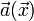$~\vec{a}(\vec{x})$, felt at any point in space,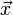$~\vec{x}$, due to the gravitational attraction of a distribution of mass,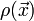$~\rho(\vec{x})$, is obtained by integrating over the accelerations exerted by each small mass element,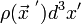$~\rho(\vec{x}^{~'}) d^3x'$, as follows:$~\vec{a}(\vec{x})$$~=$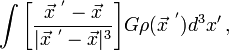$~ \int \biggl[\frac{\vec{x}^{~'} - \vec{x}}{|\vec{x}^{~'} - \vec{x}|^3}\biggr] G\rho(\vec{x}^{~'}) d^3 x' \, ,$ [BT87], p. 31, Eq. (2-2)

where,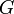$~G$ is the universal gravitational constant.

## Step 1

In the astrophysics literature, it is customary to adopt the following definition of the,

 Scalar Gravitational Potential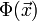$~ \Phi(\vec{x})$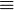$~\equiv$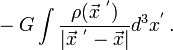$~ -G \int \frac{\rho(\vec{x}^{~'})}{|\vec{x}^{~'} - \vec{x}|} d^3x^' \, .$ [BT87], p. 31, Eq. (2-3) [EFE], §10, p. 17, Eq. (11) [T78], §4.2, p. 77, Eq. (12)

(Note:   As we have detailed in a separate discussion, throughout [EFE] Chandrasekhar adopts a different sign convention as well as a different variable name to represent the gravitational potential.) Recognizing that the gradient of the function,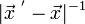$~|\vec{x}^{~'} - \vec{x}|^{-1}$, with respect to$~\vec{x}$ is,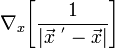$~\nabla_x \biggl[ \frac{1}{|\vec{x}^{~'} - \vec{x}|} \biggr]$$~=$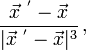$~ \frac{\vec{x}^{~'} - \vec{x}}{|\vec{x}^{~'} - \vec{x}|^3} \, ,$ [BT87], p. 31, Eq. (2-4)

and given that, in the above expression for the gravitational acceleration, the integration is taken over the volume that is identified by the primed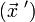$~(\vec{x}~{'})$, rather than the unprimed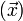$~(\vec{x})$, coordinate system, we find that we may write the gravitational acceleration as,$~\vec{a}(\vec{x})$$~=$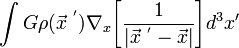$~\int G\rho(\vec{x}^{~'}) \nabla_x \biggl[ \frac{1}{|\vec{x}^{~'} - \vec{x}|} \biggr]d^3 x'$$~=$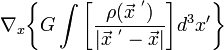$~ \nabla_x \biggl\{ G \int \biggl[ \frac{\rho(\vec{x}^{~'}) }{|\vec{x}^{~'} - \vec{x}|} \biggr]d^3 x'\biggr\}$$~=$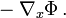$~-\nabla_x \Phi \, .$ [BT87], p. 31, Eq. (2-5)

## Step 2

Next, we realize that the divergence of the gravitational acceleration takes the form,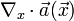$~\nabla_x \cdot \vec{a}(\vec{x})$$~=$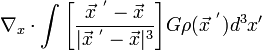$~ \nabla_x \cdot \int \biggl[\frac{\vec{x}^{~'} - \vec{x}}{|\vec{x}^{~'} - \vec{x}|^3}\biggr] G\rho(\vec{x}^{~'}) d^3 x'$$~=$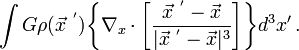$~ \int G\rho(\vec{x}^{~'}) \biggl\{ \nabla_x \cdot \biggl[\frac{\vec{x}^{~'} - \vec{x}}{|\vec{x}^{~'} - \vec{x}|^3}\biggr] \biggr\} d^3 x' \, .$ [BT87], p. 31, Eq. (2-6)

Examining the expression inside the curly braces, we find that,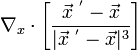$~\nabla_x \cdot \biggl[\frac{\vec{x}^{~'} - \vec{x}}{|\vec{x}^{~'} - \vec{x}|^3}\biggr]$$~=$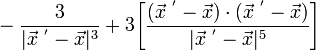$~ - \frac{3}{|\vec{x}^{~'} - \vec{x}|^3} + 3 \biggl[ \frac{ (\vec{x}^{~'} - \vec{x}) \cdot (\vec{x}^{~'} - \vec{x}) }{|\vec{x}^{~'} - \vec{x}|^5}\biggr]$

(Note:   Ostensibly, this last expression is the same as equation 2-7 of [BT87], but apparently there is a typesetting error in the BT87 publication. As printed, the denominator of the first term on the right-hand side is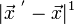$~|\vec{x}^{~'} - \vec{x}|^1$, whereas it should be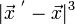$~|\vec{x}^{~'} - \vec{x}|^3$ as written here.) When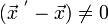$~(\vec{x}^{~'} - \vec{x}) \ne 0$, we may cancel the factor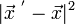$~|\vec{x}^{~'} - \vec{x}|^2$ from top and bottom of the last term in this equation to conclude that,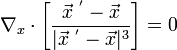$~\nabla_x \cdot \biggl[\frac{\vec{x}^{~'} - \vec{x}}{|\vec{x}^{~'} - \vec{x}|^3}\biggr] = 0$ when,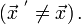$~ (\vec{x}^{~'} \ne \vec{x}) \, .$ [BT87], p. 31, Eq. (2-8)

Therefore, any contribution to the integral must come from the point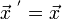$~\vec{x}^{~'} = \vec{x}$, and we may restrict the volume of integration to a small sphere … centered on this point. Since, for a sufficiently small sphere, the density will be almost constant through this volume, we can take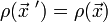$~\rho(\vec{x}~{'}) = \rho(\vec{x})$ out of the integral. Via the divergence theorem (for details, see appendix 1.B — specifically, equation 1B-42 — of [BT87]), the remaining volume integral may be converted into a surface integral over the small volume centered on the point$~\vec{x}^{~'} = \vec{x}$ and, in turn, this surface integral may be written in terms of an integral over the solid angle,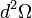$~d^2\Omega$, to give:$~\nabla_x \cdot \vec{a}(\vec{x})$$~=$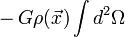$~ -G\rho(\vec{x}) \int d^2\Omega$$~=$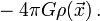$~ -4\pi G\rho(\vec{x}) \, .$ [BT87], p. 32, Eq. (2-9b)

## Step 3

Finally, combining the results of Step 1 and Step 2 gives the desired,

Poisson Equation$\nabla^2 \Phi = 4\pi G \rho$

which serves as one of the principal governing equations in our examination of the Structure, Stability, & Dynamics of Self-Gravitating Fluids.